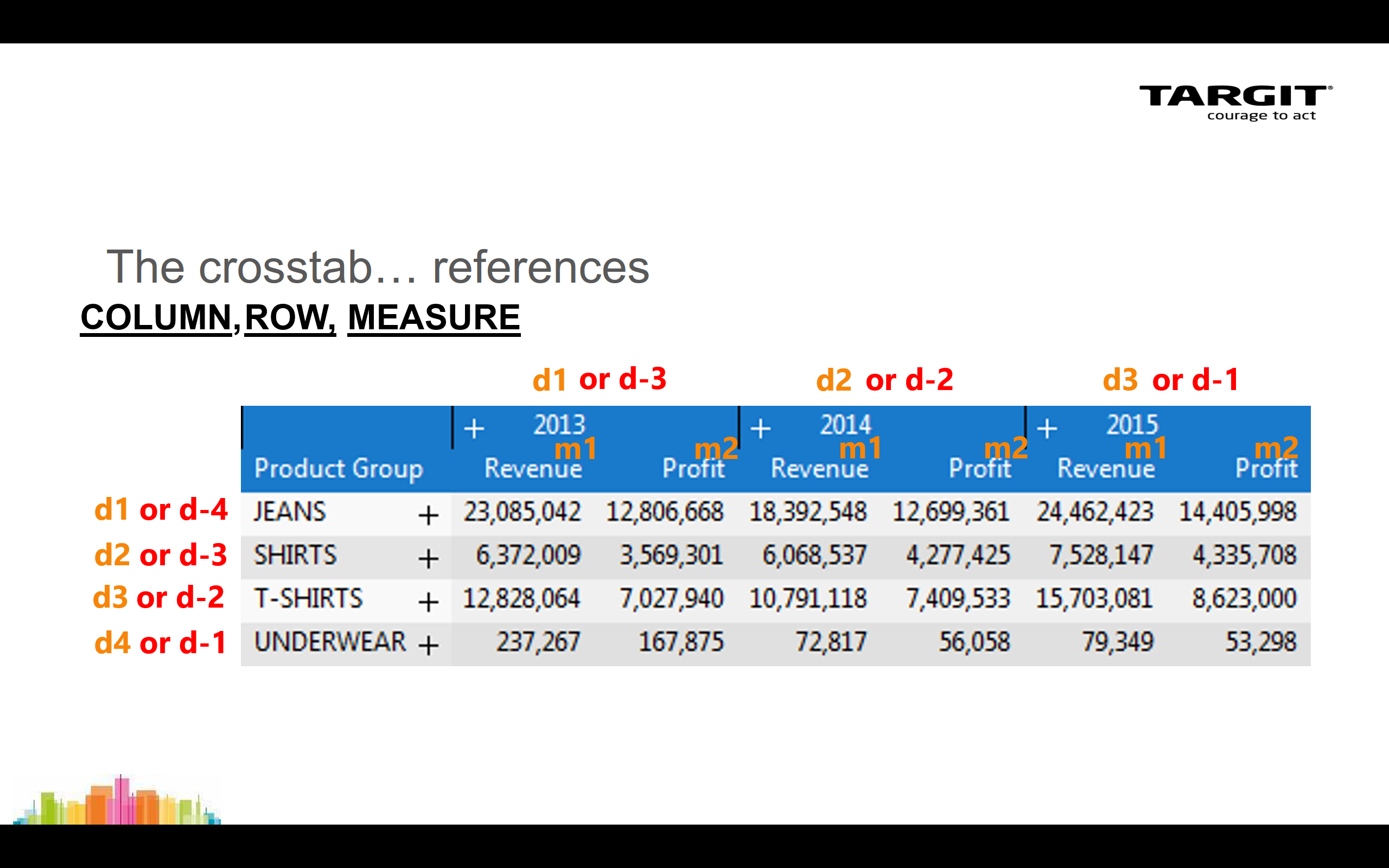##### Page tree
Skip to end of banner
Go to start of banner

# Calculations Expert Course

Skip to end of metadata
Go to start of metadata

# Calculations Expert Course

## The Syntax, explained

The syntax is about being able to refer to Columns, Rows and Measures within a crosstab.

The general syntax is then function(column, row, measure).## Simple calculations in crosstab

A couple of examples on simple calculations in a crosstab:

• "Difference" as a calculated column.
• "Average" as a calculated row.

The first calculated column inserted in this way may later be referred to as e.g. sum(c1, 0, m1).

The first calculated row inserted in this way may later be referred to as e.g. sum(0, c1, m1).

## Calculation "as a new measure"

When you insert a calculation "as a new measure" in a crosstab that already have e.g. 2 measures, m1 and m2, then the new calculation can be referred to as m3.

## Calculations in graphical charts

Calculations in graphs are not different from calculations in crosstabs. In fact, your syntax still refer to the crosstab behind tha graph.

This example will show you how to add calculations to graphs. Various average calculations, including different range references, are demonstrated.

## Named Dimension Member Reference

The Named Dimension Member Reference method is useful when you want to refer to a specific row member or a specific column member in your data set.

Sometimes a reference to eg. "the fifth row" is not what you want, because one day that will refer to one customer, but on another day - due to data changed in the meantime - that may refer to another customer.

## If-then-else statements

You can make conditional calculations on basis of your data values by use of if-then-else statements.

## The fourth syntax reference - The "Undefined"

Sometimes you will experience that your calculations return an "Undefined" result. This could happen if you are using a relative reference to something outside the current data scope.

To prevent "Undefined" from appearing, you can work with a fourth parameter in your calculation syntax.

## The Count and Allcount functions

The Count function will count all non-empty cells within the reference range.

The Allcount function will count all cells, empty and non-empty, within the reference range.

## The Visibility Modifier

When your data set in the TARGIT client is influenced by visibility option, i.e. some data have been hidden, you may need to add Visibility Modifiers, (v) or (h), to your calculations.

## The Order Modifier

When data in the TARGIT client has been sorted, eg. descending or ascending, you may need to use Order modifiers in your calculations.

In this way you can make your calculation work according to the original, unsorted order - while at the same time display data in a sorted order.

The Order modifier may also be necessary to include if you intend to sort your data according to calculated values.

## Hierarchy modifiers: Children

A multilevel hierarchy can be viewed as a tree structure with nodes and branches. Each node at a higher level may have a number of children at a lower level.

## The Siblings modifier

In a hierarchy, dimension members with a common parent node can be referred to as Siblings.

When added as a modifier to the calculation syntax, you can then start calculating eg. averages within individual groups of data in the same crosstab.

## The Level modifier

Individual levels of a hierarchical dimension can be referred to as Level 1, Level 2 etc.

In most cases, the default behavior of a TARGIT calculation, i.e. to work on the current level is what you want. But, with the Level modifier, you can focus your calculation to a specific level of a hierarchy.

## The Level modifier in a Custom Hierarchy

Custom Hierarchy is a hierarchy that you have created eg. by adding to different dimensions to the same axis of a crosstab.

The Level modifier will then need to take all dimensions into account as shown in this example.

• No labels